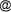## Siwaporn Pradidwong, Anand Isarasenee, Elke Pawelzik: Prediction of Mungbean Seed Longevity and Quality Using the Relationship of Seed Moisture Content and Storage Temperature

SIWAPORN PRADIDWONG1, ANAND ISARASENEE1, ELKE PAWELZIK2
1Chiang Mai University, Department of Agronomy, Thailand
2Georg-August-Universität Göttingen, Institute of Agricultural Chemistry, Germany

The prediction equation of mungbean seeds viability and quality in relation to seed moisture content and storage temperature were established. Mungbean seeds (Vigna radiata (L.) WILCZEK) ev. cultivar Chainat 72 (CN 72) with four initial seed moisture content of 7, 9, 11 and 13 percent and four storage temperature of 15, 20, 25 degree Celsius including room temperature (27-32 degree Celsius) were studies. Seed were store for 18 weeks and the assessments were done every 3 weeks. Multiple regression were analysed: standard germination test, vigour test by accelerated aging technique, seedling growth rate and electrical conductivity from seed exudate, tetrazolium technique for viability, fungi infection, insect infection, protein and carbohydrate content were investigated. The regression equation for predicting the viability were by following: dependent variable (Y), independent variables were initial seed moisture content (X1), storage temperature (X2) and period of storage (X3). The best equation resulted by seedling growth rate which Y = 26.153-0.878 (X1) + 0.147 (X1 x 3) + 0.039 (X12) - 0.007 (X12 x 3) - 0.025 (X2) - 0.558 (X3) - 0.01 (X32) (R2 = 0.9615). The others equation are electrical conductivity Y = 80.757-1.771 (X1) + 0.069 (X1 x 2) + 0.153 (X1 x 3) - 0.343 (X2) - 2.654 (X3) + 0.172 (X32) (R2 = 0.9595) and accelerated aging Y = 118.414-2.180 (X1) + 0.047 (X1 x 2) + 0.369 (X1 x 3) - 0.019 (X12 x 3) - 0.873 (X2) - 2.996 (X3) + 0.043 (X32) (R2 = 0.9119). This equation could be used to predict viability and storability of mungbean seeds under above mentioned condition, their predict result were closely to the observed values of mungbean seed qualities.

Keywords: Prediction, seed viability test, seed vigour test, storage

#### Footnotes

...PContact Address: Siwaporn Pradidwong, Chiang Mai University, Department of AgronomyChiang Mai, Thailand, e-mail: roteesyahoo.com
Andreas Deininger, September 2004